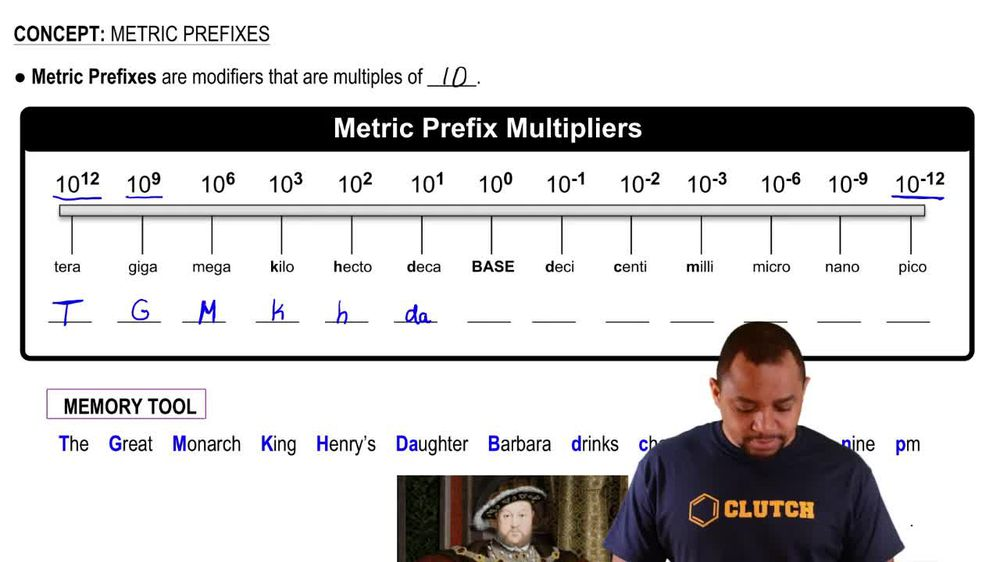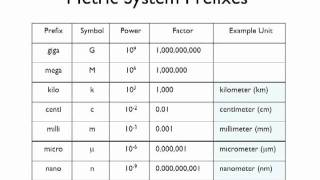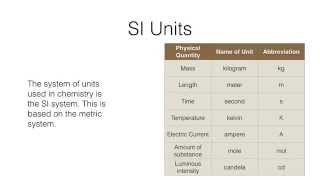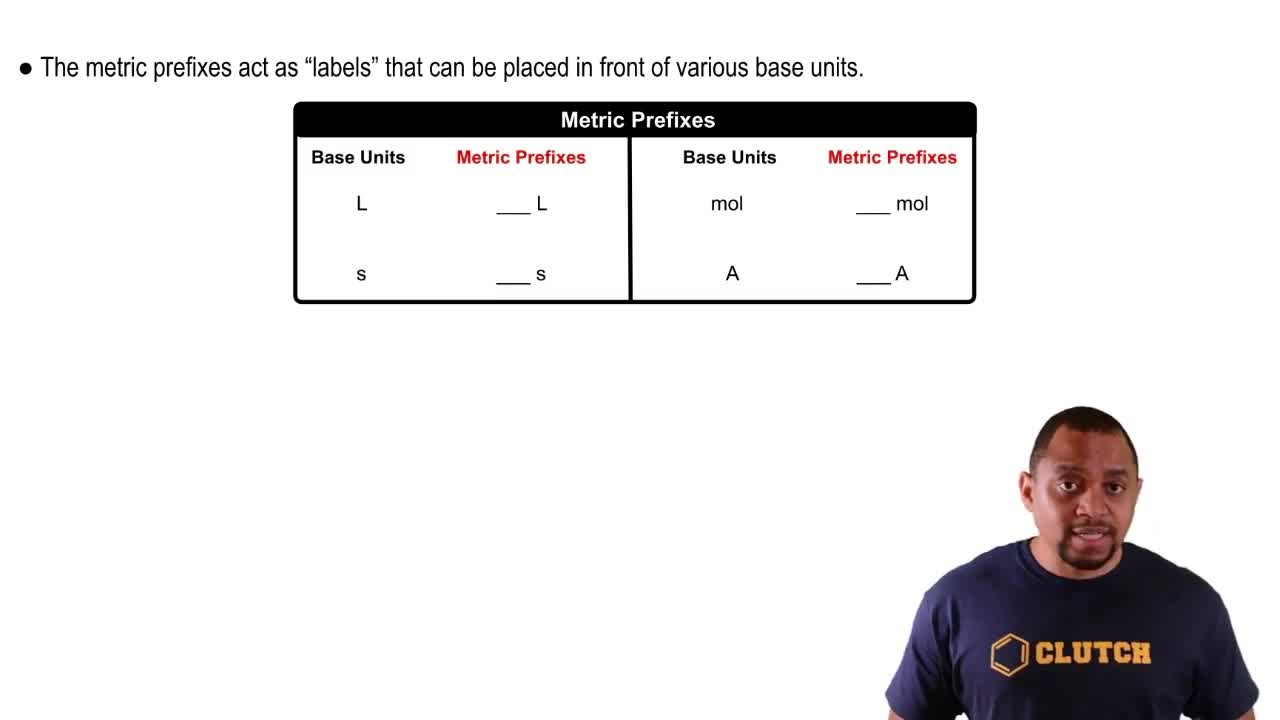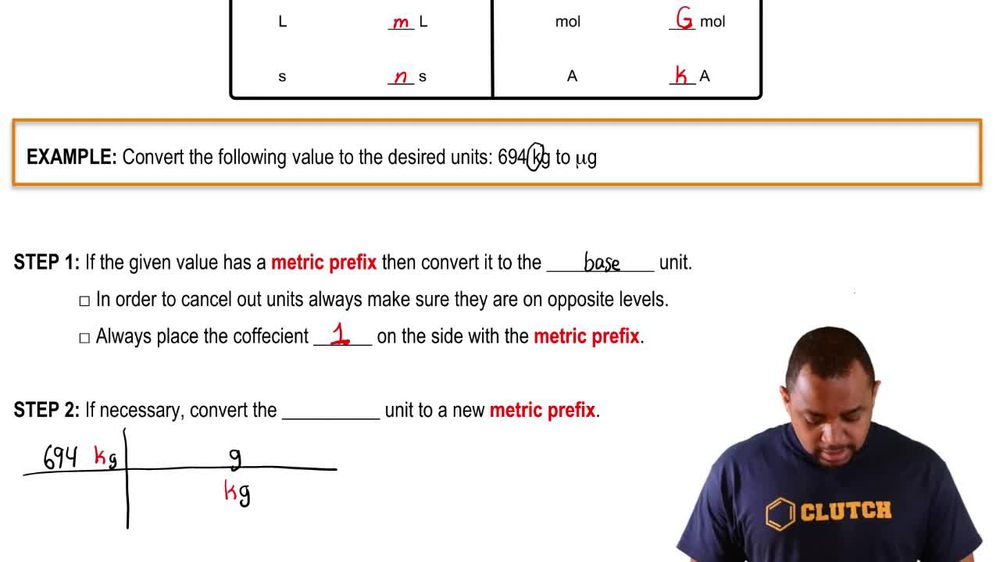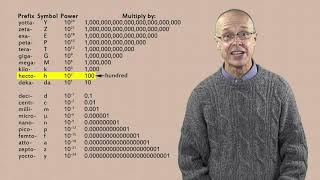Start typing, then use the up and down arrows to select an option from the list.
1. 1. Intro to General Chemistry2. Metric Prefixes
Problem

# Which quantity in each of the following pairs is smaller? (c) 2.9 GA or 3.1 * 1015 mA

Relevant Solution1m
Play a video:
Hello everyone today, We are being given the following problem, determine a larger quantity between the following pair. 2.9 times 10 to the negative fourth gigawatts or 7. times 10 to the third kilowatts. So the first thing that we want to do If you want to take our gigawatts are 2.9 times 10. It's the negative fourth gigawatts. We want to transform this into regular watts. And so by doing that we can use the conversion factor that one gigawatt is equal to 10 to the 9th watts. And by doing we can also further this by converting this watts into kilowatts and comparing that with the kilowatts we already have. So we're going to use the further conversion factor that one kilowatts, is it go to 10 to the third watts. And so when we cancel units out, we're left with 2.9 times 10 to the second kilowatts. And when we compare that with our original one, r 7.1 times 10 to the third kilowatts. That is going to be a greater value than our original or the one that we just converted to. And so therefore our 7.1 times 10 to the third kilowatts is going to be the larger value between the two. And with that we've answered the question, I hope this helped until next time